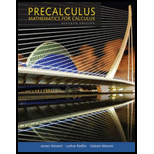Chapter 10.3, Problem 22EPrecalculus: Mathematics for Calcu...

7th Edition
James Stewart + 2 others
ISBN: 9781305071759

Solutions

Chapter
SectionPrecalculus: Mathematics for Calcu...

7th Edition
James Stewart + 2 others
ISBN: 9781305071759
Textbook Problem

Elementary Row Operations Perform the indicated elementary row operation.22. [ − 5 2 − 3 3 10 − 3 1 − 20 − 1 3 1 8 ] Add 2 times Row 1 to Row 2.

To determine

The row operations in the given matrix.

Explanation

The given matrix is,



Apply elementary row transformation, by adding 2 times Row1 to Row2.

=[523310103+41620

Still sussing out bartleby?

Check out a sample textbook solution.

See a sample solution

The Solution to Your Study Problems

Bartleby provides explanations to thousands of textbook problems written by our experts, many with advanced degrees!

Get Started

Evaluate the integral. 0/4sectand

Single Variable Calculus: Early Transcendentals

Solving an Equation In Exercises 722, solve for x. ex=5

Calculus: Early Transcendental Functions (MindTap Course List)

For the area of the shaded region, which integral form, dx or dy, should be used? a) dx b) dy c) Either...

Study Guide for Stewart's Single Variable Calculus: Early Transcendentals, 8th